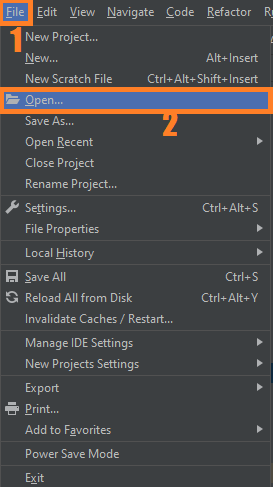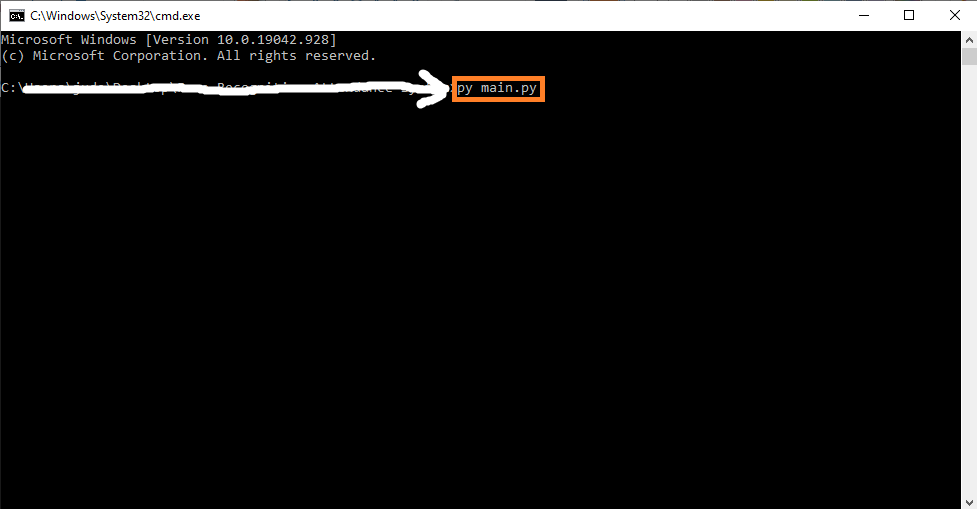# Real-Time Drowsiness Detection OpenCV Python With Source Code

## Real-Time Drowsiness Detection OpenCV Python With Source Code

The Real-Time Drowsiness Detection OpenCV Python was developed using Python OpenCV, This Drowsiness Detection is a safety technology that can prevent accidents that are caused by drivers who fell asleep while driving.

A Drowsiness Detection OpenCV Python project, we will be using OpenCV for gathering the images from webcam and feed them into a Deep Learning model which will classify whether the person’s eyes are ‘Open’ or ‘Closed’.

By the way if you are new to python programming and you don’t know what would be the the Python IDE to use, I have here a list of Best Python IDE for Windows, Linux, Mac OS that will suit for you. I also have here How to Download and Install Latest Version of Python on Windows.

To start executing Real-Time Drowsiness Detection OpenCV Python With Source Code, make sure that you have installed Python 3.9 and PyCharm in your computer.

## Real-Time Drowsiness Detection OpenCV Python With Source Code : Steps on how to run the project

Time needed: 5 minutes

These are the steps on how to run Real-Time Drowsiness Detection OpenCV Python With Source Code

First, download the given source code below and unzip the source code.• Step 2: Import the project to your PyCharm IDE.• Step 3: Run the project.

last, run the project with the command “py main.py”## Installed Libraries

```from scipy.spatial import distance
from imutils import face_utils
import imutils
import dlib
import cv2```

## Complete Source Code

```from scipy.spatial import distance
from imutils import face_utils
import imutils
import dlib
import cv2

def eye_aspect_ratio(eye):
A = distance.euclidean(eye, eye)
B = distance.euclidean(eye, eye)
C = distance.euclidean(eye, eye)
ear = (A + B) / (2.0 * C)
return ear

thresh = 0.25
frame_check = 20
detect = dlib.get_frontal_face_detector()
predict = dlib.shape_predictor(".\shape_predictor_68_face_landmarks.dat")# Dat file is the crux of the code

(lStart, lEnd) = face_utils.FACIAL_LANDMARKS_68_IDXS["left_eye"]
(rStart, rEnd) = face_utils.FACIAL_LANDMARKS_68_IDXS["right_eye"]
cap=cv2.VideoCapture(0)
flag=0
while True:
frame = imutils.resize(frame, width=450)
gray = cv2.cvtColor(frame, cv2.COLOR_BGR2GRAY)
subjects = detect(gray, 0)
for subject in subjects:
shape = predict(gray, subject)
shape = face_utils.shape_to_np(shape)#converting to NumPy Array
leftEye = shape[lStart:lEnd]
rightEye = shape[rStart:rEnd]
leftEAR = eye_aspect_ratio(leftEye)
rightEAR = eye_aspect_ratio(rightEye)
ear = (leftEAR + rightEAR) / 2.0
leftEyeHull = cv2.convexHull(leftEye)
rightEyeHull = cv2.convexHull(rightEye)
cv2.drawContours(frame, [leftEyeHull], -1, (0, 255, 0), 1)
cv2.drawContours(frame, [rightEyeHull], -1, (0, 255, 0), 1)
if ear < thresh:
flag += 1
print (flag)
if flag >= frame_check:
cv2.FONT_HERSHEY_SIMPLEX, 0.7, (0, 0, 255), 2)
cv2.FONT_HERSHEY_SIMPLEX, 0.7, (0, 0, 255), 2)
#print ("Drowsy")
else:
flag = 0
cv2.imshow("Frame", frame)
key = cv2.waitKey(1) & 0xFF
if key == ord("q"):
break
cv2.destroyAllWindows()
cap.stop()
```